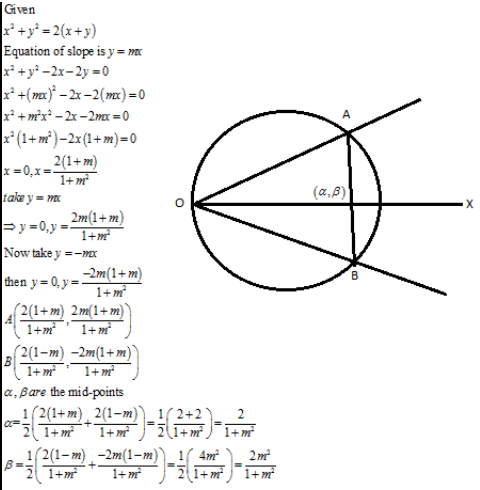# the equation of the locus of the middle point of a chord of the circle x^2 + y^2=2(x+y) such that the pair of lines joining the origin to the point of intersection of the chord and the circle are equally inclined to the x-axis is:   (a)x+y=2 (b)x-y=2 (c)2x-y=1 (d)none of these   I WILL B VERY THANKFUL TO U IF U GIVE AN ELABORATED SOLUTION...THANKUSaurabh Koranglekar